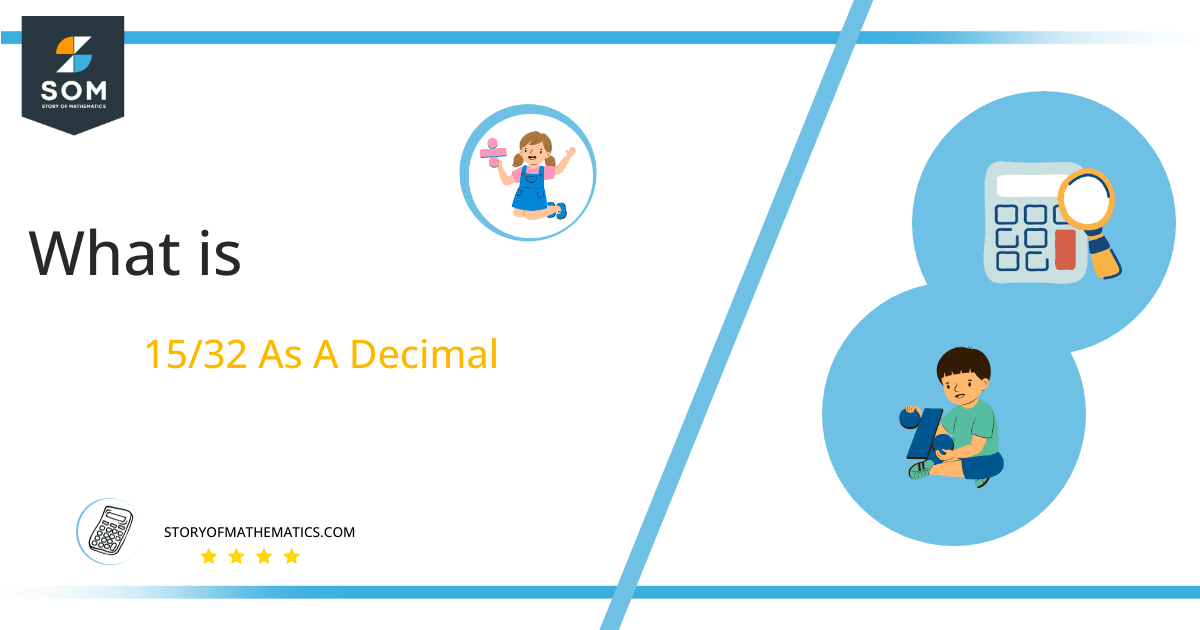# What Is 15/32 as a Decimal + Solution With Free Steps

The fraction 15/32 as a decimal is equal to 0.468.

A Fraction is a mathematical expression used to divide an object or number into a certain number of equal pieces.  The numerator and denominator, which are two parts of the fraction, are divided to solve it and get the answer as a decimal value.

Here, we are more interested in the division types that result in a Decimal value, as this can be expressed as a Fraction. We see fractions as a way of showing two numbers having the operation of Division between them that result in a value that lies between two Integers.Now, we introduce the method used to solve said fraction to decimal conversion, called Long Division which we will discuss in detail moving forward. So, let’s go through the Solution of fraction 15/32.

## Solution

First, we convert the fraction components i.e., the numerator and the denominator, and transform them into the division constituents i.e., the Dividend and the Divisor respectively.

This can be seen done as follows:

Dividend = 15

Divisor = 32

Now, we introduce the most important quantity in our process of division, this is the Quotient. The value represents the Solution to our division, and can be expressed as having the following relationship with the Division constituents:

Quotient = Dividend $\div$ Divisor = 15 $\div$ 32

This is when we go through the Long Division solution to our problem. The mathematical steps of this division are shown in figure 1.Figure 1

## 15/32 Long Division Method

We start solving a problem using the Long Division Method by first taking apart the division’s components and comparing them. As we have 15, and 32 we can see how 15 is Smaller than 32, and to solve this division we require that 15 be Bigger than 32.

This is done by multiplying the dividend by 10 and checking whether it is bigger than the divisor or not and if it is then we calculate the Multiple of the divisor which is closest to the dividend and subtract it from the Dividend. This produces the Remainder which we then use as the dividend later.

Now, we begin solving for our dividend 15, which after getting multiplied by 10 becomes 150.

We take this 150 and divide it by 32, this can be seen done as follows:

150 $\div$ 32 $\approx$ 4

Where:

32 x 4 = 128

This will lead to the generation of a Remainder equal to 150 – 128 = 22, now this means we have to repeat the process by Converting the 22 into 220 and solving for that:

220 $\div$ 32 $\approx$ 6

Where:

32 x 6 = 192

This, therefore, produces another remainder which is equal to 220 – 192 = 28. Now we must solve this problem to Third Decimal Place for accuracy, so we repeat the process with dividend 280.

280 $\div$ 32 $\approx$ 8

Where:

32 x 8 = 256

Finally, we have a Quotient generated after combining the three pieces of it as 0.468 = z, with a Remainder equal to 24.Images/mathematical drawings are created with GeoGebra.